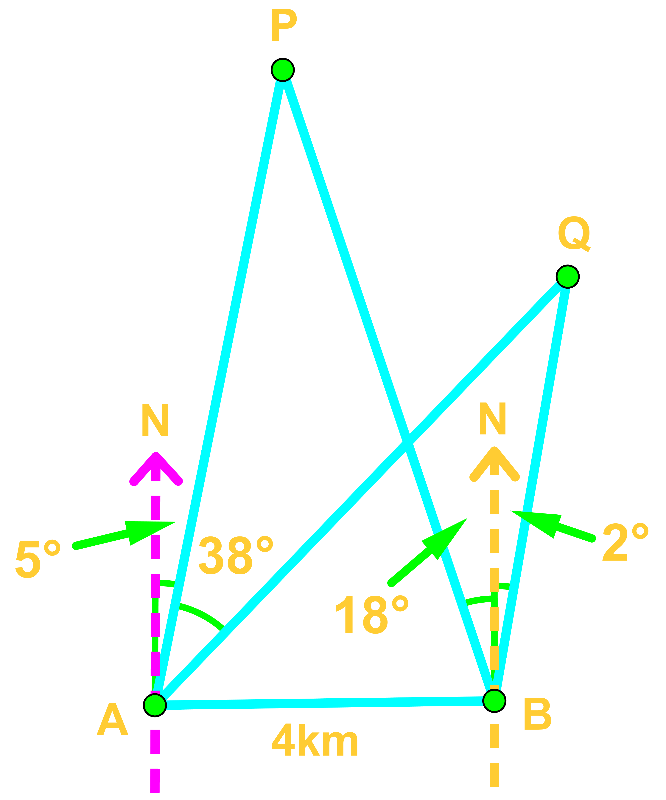# Bearings and direction word problems

### Bearings and direction word problems

#### Lessons

Theorems that are useful:

Pythagorean Theorem: $a^{2} + b^{2} = c^{2}$

Trig ratio: $\sin \theta = \frac{O}{H}$

$\cos \theta = \frac{A}{H}$

$\tan \theta = \frac{O}{A}$

Law of sine: $\frac{a}{\sin A} = \frac{b}{\sin B} = \frac{c}{\sin C}$

Law of cosine: $c^{2} = a^{2} + b^{2} - 2ab \cos C$

• Introduction
Introduction to Bearings and Direction Word Problems

• 1.
Evaluate A Bearings Word Problem Using Trigonometric Ratios

Charlie leaves home for a bike ride, heading 040°T for 5km.

a)
How far north or south is Charlie from its starting point?

b)
How far east or west is Charlie from its starting point?

• 2.
Solve A Bearings Word Problem Using the Law of Cosine

A camping group made a return journey from their base camp. From the camp, they first travelled 120°T for 3km. Then they travelled 210°T for 9km. Determine the direction and distance they need to travel if they want to return to the base camp now.

• 3.
Analyze A Bearings Word Problem Using Trigonometric Ratios and the Law of Cosine

Melody and April go to the same school. Melody's home is 3.5km with a bearing of S16°W from school whilst April's home is 2.4km with a bearing of N42°E from school. How far away are their homes from each other?

• 4.
Triangulate the Location of an Earthquake

Radar X detected an earthquake N55°E of it. 16km due east of Radar X, Radar Y detected the same earthquake N14°W of it.

a)
Determine the earthquake from Radar X and Y.

b)
Which Radar is closer to the earthquake?

• 5.
Estimate the Height of an Object

A plane is sighted by Tom and Mary at bearings 028°T and 012°T respectively. If they are 2km away from each other, how high is the plane?

• 6.
Applying Law of Sine and Law of Cosine

Consider the following diagram.Find the distance between P and Q.